### Eyes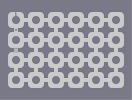Hover over the thumbnail for a full-size version.

Author jalle7 author:jalle7 incomplete unrated 2006-02-04 3 more votes required for a rating. \$Eyes#jalle7#none#1111111111111111111111111900619006190061900611110;<0Q0;<0Q0;<0Q0;<011110:=0O0:=0O0:=0O0:=01111800718007180071800711111PN111PN111PN111PN11111900619006190061900611110;<0Q0;<0Q0;<0Q0;<011110:=0O0:=0O0:=0O0:=01111800718007180071800711111PN111PN111PN111PN11111900619006190061900611110;<0Q0;<0Q0;<0Q0;<011110:=0O0:=0O0:=0O0:=01111800718007180071800711111PN111PN111PN111PN11111900619006190061900611110;<0Q0;<0Q0;<0Q0;<011110:=0O0:=0O0:=0O0:=01111800718007180071800711111PN111PN111PN111PN11111900619006190061900611110;<0Q0;<0Q0;<0Q0;<011110:=0O0:=0O0:=0O0:=01111800718007180071800711111PN111PN111PN111PN11111900619006190061900611110;<0Q0;<0Q0;<0Q0;<011110:=0O0:=0O0:=0O0:=0111180071800718007180071111111111111111111111111|5^96,84!11^96,300,96,180# Free tileset. I dont know what to do with it, but it looks cool

## Other maps by this author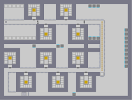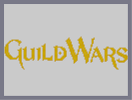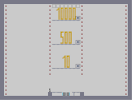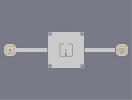The Ten Traps Guild Wars Power Jump Escape